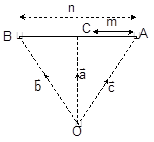internal and external divison of vectorsRaj Kumar
11/09/2018 00

If A and B are two points with position vectors a and b respectively and C is a point which divides AB internally in the ratio m: n, then the position vector of C is given by OC = (mb + na)/ (m + n). This is termed as internal division.which is shown by below figure.

If A and B are two points with position vectors a and b respectively and C is a point which divides AB externally in the ratio m : n, then the position vector of C is given by OC = (mb - na)/ (m-n)0 Dislike
Follow 2Other Lessons for You

Vectors and scaler
Vector is that quantity that has direction and magnitude for example force, velocity, acceleration and displacement The scaler is that quantity which has the only magnitude for example speed, volume,...Geometric Progression
If a is the first term and r is the common ratio of the geometric progression, then its nth term is given by an = arn-1 The sum Sn of the first n terms of the G.P. is given by Sn = a (rn – 1)/...Notes on Arithmetic progression
If ‘a’ is the first term and ‘d’ is the common difference of the arithmetic progression, then its nth term is given by an = a+(n-1)d The sum, Sn of the first ‘n’...Notes on Harmonic progression
Let a, b and c form an H.P. Then 1/a, 1/b and 1/c form an A.P. If a, b and c are in H.P. then 2/b = 1/a + 1/c, which can be simplified as b = 2ac/(a+c) If a and b are two non-zero numbers...Cordinate Axis retangular to polar and polar to rectangular Conversion
The system of coordinates is known as the Cartesian System of Coordinates. It is so called because this system was first introduced by the philosopher Des Cartes. There are other systems of coordinates...Looking to Learn?

Find best Tutors and Coaching Centers near you on UrbanPro.

FIND NOW

Are you a Tutor or Training Institute?

Join UrbanPro Today to find students near you